Reach Us+44-1474-556909
Common Fixed Points of Generalized andalpha;-andpsi;-contractive Type Self-mappings and Multivalued Mappings | OMICS International
Journal of Applied & Computational Mathematics
All submissions of the EM system will be redirected to Online Manuscript Submission System. Authors are requested to submit articles directly to Online Manuscript Submission System of respective journal.

# Common Fixed Points of Generalized α-ψ-contractive Type Self-mappings and Multivalued Mappings

Department of Mathematics, Marand Branch, Islamic Azad University, Marand, Iraq

*Corresponding Author:
Department of Mathematics, Marand Branch
Tel:
+98214486 5179;
E-mail: [email protected]

Received Date: January 19, 2016; Accepted Date: February 05, 2016; Published Date: February 10, 2016

Citation: Mohammadi B (2016) Common Fixed Points of Generalized α-ψ-contractive Type Self-mappings and Multivalued Mappings. J Appl Computat Math 5:287. doi:10.4172/2168-9679.1000287

Visit for more related articles at Journal of Applied & Computational Mathematics

#### Abstract

In this paper, mutivated by the recent work of samet et al. (Fixed point theorems for α-ψ-contractive type mappings, Nonlinear Anal. we give some new results on existence of common fixed points for a pair of generalized α-ψ-contractive self-mappings and multivalued mappings. This results extend and improve many existing results in the literature. Some examples are given to illustrate the results.

#### Keywords

Common fixed point; Generalized α-ψ-contractive; Selfmappings; Multivalued mappings

#### Introduction

Recently, some results on existing of common fixed point for a pair of mappings or multifunctions have been given. In 2007, Zhang  defined a new generalized contractive type condition for a pair of mappings in metric spaces. Let A∈(0,+∞] and :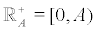. Denote by ℑ[0,A) the collection of all functions :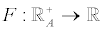satisfing the following conditions:

(i) F(0)=0 and F(t)>0 for each t∈(0,A),

(ii) F is nondecreasing on A +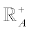,

(iii) F is continuous.

From Zhang  we know that for any F∈ℑ[0,A), limn→∞F(εn)=0,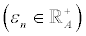implies limn→∞εn=0. Denote by Ψ[0,A) the family of all functions :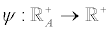which is nondecreasing and right upper semicontinuous such that limn→∞εΨn(t)=0 for each t∈(0,A). It is easy to see that for any ψ∈Ψ[0,A) we have ψ(0)=0 and ψ(t)<t for each t∈(0,A).

Regarding the above notations, Zhang  proved the following theorem on existing of common fixed point for a pair of mappings.

Theorem 1: Let (X,d) be a complete metric space and let D = sup{d(x, y) | x, y∈ X} . Set A>D if D<∞ and A=D if D=∞. Suppose that T,S:X→X are two mappings,F∈ℑ[0,A) and ψ∈Ψ[0,F(A-)) satisfing

F(d(Tx, Sy)) ≤ψ (F(M(x, y))) for each x, y∈ X

where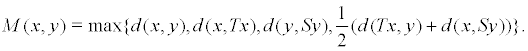Then T and S have a unique common fixed point in X. Moreover, for each x0∈X, the iterated sequence {xn} with x2n+1=Tx2n and x2n+2= Sx2n+1 converges to the common fixed point of T and S.

Denote by Ψ the family of all nondecreasing functions ψ:[0,+∞)→[0,+ ∞) such that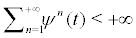for all t>0. Let (X,d) be a metric space and α: X×X→[0,∞) be a mapping. A mapping T:X→X is called α-admissible whenever α(x,y)≥1 implies α(Tx,Ty)≥1. In 2011, Samet and Vetro  introduced a new type of contraction to a mapping T:X→X, called α-ψ- contractive mappings, that is, α(x,y)d(Tx,Ty)≤ψ(d(x,y)) for all x,y∈X and proved the following theorem.

Theorem 2: Let (X,d) be a complete metric space, α:X×X→[0,+∞) a function, ψ∈Ψ and T:X→X be an α-ψ-contractive mapping such that the following assertions hold :

(ii) There exists x0∈X such that α(x0,Tx0)≥1,

(iii) T is continuous or for any sequence {xn} in X that α(xn,xn+1)≥1 for all n and xn→x, we have α(xn,x)≥1 for all n.

Then, T has a fixed point in X.

This result generalized and improved many existing fixed point results for a mapping defined on a complete metric space (X,d), in particular the famuous Banach contraction principle. Also, the authors proved that adding the following condition to the conditions of above theorem, the fixed point is unique.

(H):For any x,y∈X there exists z∈X such that α(x,z) ≥ 1 and α(y,z) ≥ 1.

Let (X,d) be a metric space and let CB(X) denote the set of all nonempty closed bounded subsets of X. Let H be the Hausdorff metric on CB(X) with respect to the metric d, that is,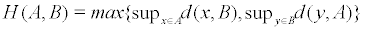for all A,B∈CB(X) where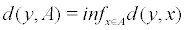. Let T,S:X→2x be a pair of multi-valued mappings. It is called that x is a common fixed point of T and S if x∈Tx and x∈Sx. In 2010, Rouhani and Moradi  proved the following common fixed point result for multi-valued mappings.

Theorem 3: Let (X,d) be a complete metric space and let T,S:X→CB(X) be two multivalued mappings such that

H(Tx, Sy) ≤αM(x, y)

for all x,y∈X where 0 ≤ α<1 andThen, T and S have a common fixed point in X.

On the other hand in 2013, Beg et al. introduced common fixed point results for multivalued mappings defined on a metric space endowed with a graph . In this paper combining ideas of the above mentioned literature we give some new results on common fixed points of α-ψ-contractive self-mappings and multivalued mappings [5-8].

Results

Now we give the main results of this study. Firstly we give some definitions.

Definition 1: Let (X,d) be a metric space and α:X×X→[0,∞) be a mapping. We say that α is symmetric if α(x,y) ≥ 1 implies α(y,x) ≥ 1. Also, it is called that α is transitive if α(x,y) ≥ 1 and α(y,z) ≥ 1 implies α(x,z) ≥ 1.

Definition 2: Let (X,d) be a metric space and T,S:X→X be two mappings. We say that the ordered pair (T,S) is α-admissible whenever α(x,y) ≥ 1 implies α(Tx,Sy) ≥ 1.

Theorem 4: Let (X,d) be a complete metric space and let D = sup{d(x, y) | x, y∈ X} . Set A>D if D<∞ and A=D if D=∞. Suppose α:X×X→[0,+∞) be a symmetric and transitive function, F∈ℑ[0,A), ψ∈Ψ[0,F(A-)) and T,S:X→X are two mappings satisfingwhereMoreover, let the following assertions hold:

(ii) There exists x0∈X such that α(x0,Tx0) ≥ 1,

(iii) For any sequence {xn} in X that α(xn,xn+1) ≥ 1 for all n and xn→x, then α(xn,x) ≥ 1 for all n

Then, T and S have a common fixed point in X.

Proof: Define an iterated sequence {xn} in X with x2n+1=Tx2n and x2n+2=Sx2n+1. Now α(x0,x1)=α(x0,Tx0) ≥ 1. Since (T,S) is α-admissible, then (x1,x2)=α(Tx0,Sx1) ≥ 1. Since α is symmetric, then (x2,x1)≥1. So, α(x3,x2)=α(Tx2,Sx1) ≥ 1. Continuing this process, we see that α(xn,xn+1) ≥ 1 for all n. Now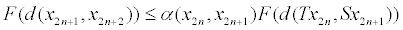(1)

(F(M(x_2n,x_2n+1))).

But we have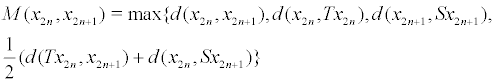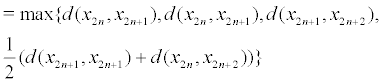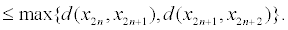If we have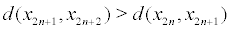, then from (1) we will have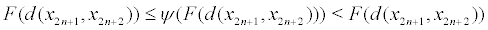which is a contradiction. So, we can only have the case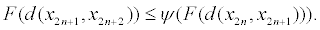(2)

Also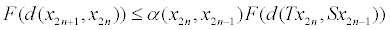(3)

(F(M(x_2n,x_2n-1))).

But we have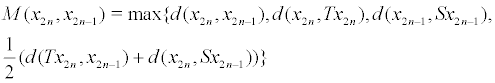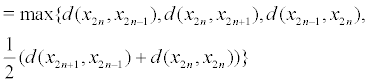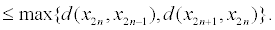If we have d(x2n+1,x2n)>d(x2n,x2n-1), then from (3) we will have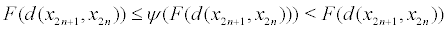which is a contradiction. So, we can only have the case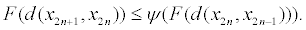(4)

From (2) and (4) we see that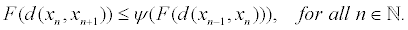(5)

From (5) we get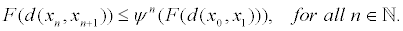Tending n to ∞, we obtain F(d(xn,xn+1))→0. Hence, d(xn,xn+1)→0 as n→∞. Since α is symmetric and transitive, hence we have α(xn,xm)≥1 and α(xm,xn)≥1 for all n, m∈N which n<m. Now showing that {xn} is a cauchy sequence is comletely similar to theorem 1 in Zhang’s . From completeness of (X,d), there exists x∈X such that xn→x.

Next, we shall show that Tx=x and Sx=x. At first, we have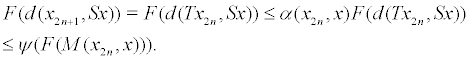(6)

ButTending n to ∞, we obtain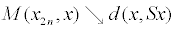. Now tending n to ∞ in (6) and using continuity of F and right upper semi-continuoty of ψ, we get F(d(x, Sx)) ≤ψ (F(d(x, Sx))) which implies F(d(x,Sx))=0 and so d(x,Sx)=0. Hence, Sx=x. On the other hand,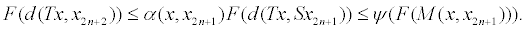(7)

But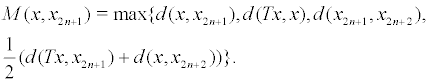Tending n to ∞, we obtain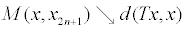. Tending n to ∞ in (7), we obtain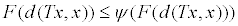which implies F(d(Tx,x))=0 and so d(Tx,x)=0. Hence, Tx=x.

Example 1: Let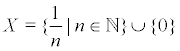with the usual metric d(x, y) =| x − y | . Obviously (X,d) is complete and D=1. Let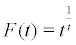on [0,e) and F(0)=0, then F∈ℑ[0,A) where A=e>D. Let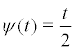. Then,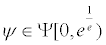. Suppose T,S:X→X be defined by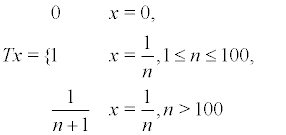and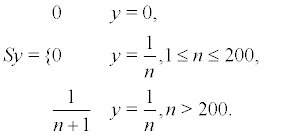Also, define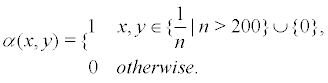Now let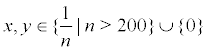If x=0 and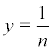where n>200, then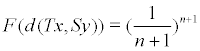and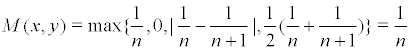Hence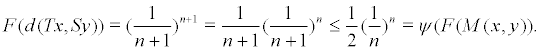Similarly, if y=0 and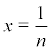where n>200, thenand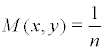and hence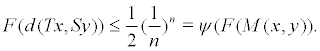If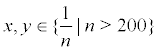, then putand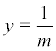where n,m>200, then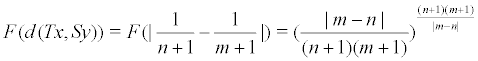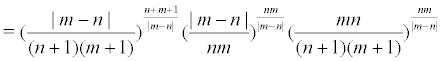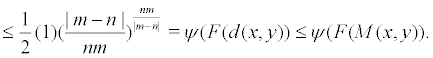By definition of α, we see that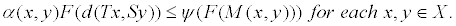Also, if we put x0=0, then α(x0,Tx0)=α(0,0)=1. Obviously, α is symmetric and transitive, (T,S) is α-admissible and the condition (iii) of theorem 4 holds. Hence, by the theorem we can say that T and S have a common fixed point in X. Here T0=S0=0.

Note that in the above example we can not apply theorem 1. To see this, put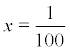and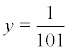. Then, F(d(Tx,Sy))=(1)1=1 and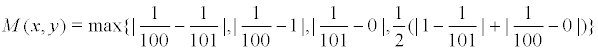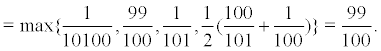We see that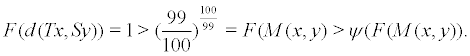Hence we can not use theorem 1. Note that theorem 4 is a generalization of theorem 1, because having the contraction condition of theorem 1 it is sufficient to put α(x,y)=1 for each x,y∈X. Then, all of the conditions of theorem 4 are holded. So by the theorem, T and S have a common fixed point in X. Also, the above example shows that this generalization is real [9-11].

Also, we can not apply the contraction α (x, y)d(Tx, Sy)) ≤ cM(x, y) for some c∈[0,1). To see this, putand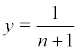where n>200. Then,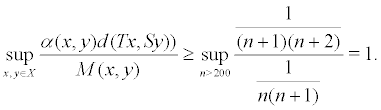Let φ:[0,A)→[0,∞) be a Lebesgue integrable function which is summable on each compact subset of [0,A) such that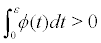for ach ε∈(0,A). Then, we have also the following result to integral type version of α-ψ-contraction for a pair of mappings T and S.

Corollary 1: Let (X,d) be a complete metric space and let D = sup{d(x, y) | x, y∈ X} . Set A>D if D<∞ and A=D if D=∞. Suppose α:X×X→[0,+∞) be a symmetric and transitive function,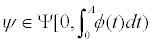and T,S:X→X are two mappings satisfing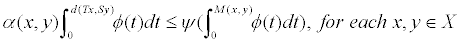whereMoreover, let the following assertions hold:

(ii) There exists x0∈X such that α(x0,Tx0)≥1,

(iii) For any sequence {xn} in X that α(xn,xn+1)≥1 for all n and xn→x, then α(xn,x)≥1 for all n.

Then, T and S have a common fixed point in X.

In what follows, we introduce the common fixed point results for a pair of multivalued mappings.

Definition 3: Let (X,d) be a metric space, α:X×X→[0,+∞) be a function and T,S:XCB(X) are two multivalued mappings. We say that the ordered pair (T,S) is α-admissible whenever α(x,y)≥1 implies α(u,v)≥1 for each u∈Tx,v∈Sy.

Theorem 5: Let (X,d) be a complete metric space and ψ∈Ψ be a strictly increasing and right upper semi-continuous function. Suppose α:X×X→[0,+∞) be a symmetric function and T,S:X→CB(X) are two multivalued mappings satisfing

α (x, y)H(Tx, Sy) ≤ψ (M(x, y)), for each x, y∈ X

whereMoreover, let the following assertions hold:

(ii) There exists x0∈x and x1∈Tx0 such that α(x0,x1)≥1,

(iii) For any sequence {xn} in X that α(xn,xn+1)≥1 for all n and xn→x, then α(xn,x)≥1 for all n.

Then, T and S have a common fixed point in X.

Proof. Obviously, M(x,y)=0 if and only if x=y is a common fixed point of T and S. Hence, we may assume that M(x0,x1)>0. Now α(x0,x1)≥1 implies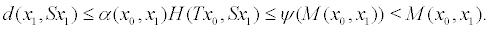(8)

But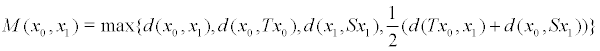≤ max{d(x , x ), d(Tx , x )}.

If d(x1,Sx1)>d(x0,x1), then from (8), we get d(x1,Sx1)<d(x1,Sx1) which is a contradiction. Hence, we have only d(x0,x1)≥d(x1,Sx1) and so from (8), we have d(x1,Sx1)<d(x0,x1). Hence, there exists x2Sx1 such that d(x1,x2)<d(x0,x1). Since (T,S) is α-admissible, hence (x1,x2)≥1 and since α is symmetric, then α(x2,x1)≥1. Now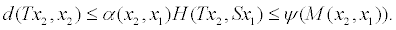(9)

But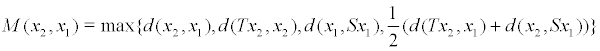≤ max{d(x , x ), d(Tx , x )}.

If d(Tx2,x2)>d(x2,x1), then from (9), we obtain d(Tx2,x2)≤ψ(d(Tx2,x2)) which is a contradiction (note that d(Tx2,x2)>d(x2,x1)≥0). Hence, we have only d(x2,x1)≥d(Tx2,x2) and so from (9), d(Tx2,x2)≤ ψ(d(x2,x1))<ψ(d(x0,x1)). Hence, there exists x3∈Tx2 such that d(x3,x2)<ψ(d(x0,x1)). Since (T,S) is α-admissible, so (x3,x2)≥1 and since α is symmetric, hence α(x2,x3)≥1. Continuing this process, we obtain a sequence {xn} in X such that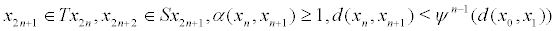for all n∈N. From triangle inequality, we conclude that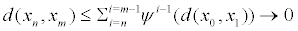. Hence, {xn} is a cauchy sequence. From completeness of (X,d), there exists x∈X such that xn→x.

Now we shall show that x∈Tx and x∈Sx. At first, we have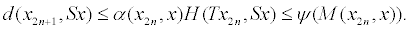(10)

But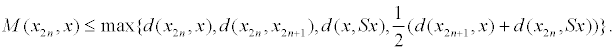Tending n to ∞, we obtain. Now tending n to ∞ in (10) and using right upper semi-continuoty of ψ, we get d(x,Sx)≤ψ(d(x,Sx)) which implies d(x,Sx)=0. Hence, x∈Sx. On the other hand,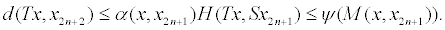(11)

But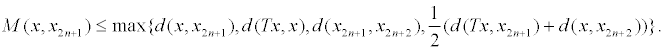Tending n to ∞, we obtain d(x, y) =| x − y | . Also tending n to ∞ in (11), we obtain d(Tx,x)≤ψ(d(Tx,x)) which implies d(Tx,x)=0 and so d(Tx,x)=0. Hence, x∈Tx.

Example 2: Let X=[0,∞) with the usual metric d(x, y) =| x − y | . Obviously, (X,d) is complete. Letand suppose T,S:X→CB(X) be defined by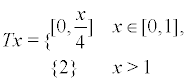and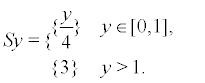Also define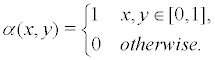Obviously, α is symmetric. If x,y∈[0,1], then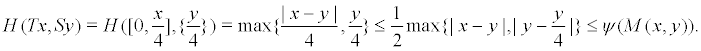Hence by definition of α, we have (x,y)H(Tx,Sy)≤ψ(M(x,y)) for all x,y∈X. It is easy to check that all of other conditions of theorem 5 hold. Hence by the theorem, T and S have a common fixed point in X. In fact, 0∈T0 and 0S0. Note that in the above example we can not apply theorem in Rouhani’s . To see this, put x=2 and y=1. Then,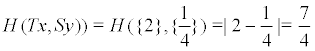and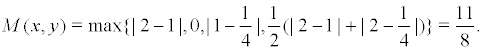We see that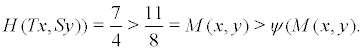Note that theorem 5 is a generalization in Rouhani’s  because having the contraction condition in Rouhani’s  it is sufficient to put α(x,y)=1 for each x,y∈X. Then, we will have all of the conditions of theorem 5 holded. So, by the theorem the multivalued mappings T and S have a common fixed point in X. Also, example 2 shows that this generalization is real.

Also, note that we can not use theorem 4 in Beg and Butt  for example 2. Since if we get E(G) = {(x, y) | x, y∈[0,1]} and choose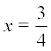and y=1, then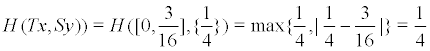and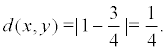We see that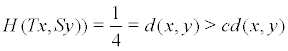for any 0≤c<1. On the other hand, theorem 2.3 is a generalization of theorem 4 in Beg and Butt  because having the contraction condition of theorem 4 in Beg and Butt  it is sufficient to define α(x,y)=1 if (x,y)∈E(G) and 0 otherwise. Example 2 shows that this generalization is real.

#### Acknowledgement

This study was supported by Marand Branch, Islamic Azad University, Iraq.

#### References

Select your language of interest to view the total content in your interested language

### Article Usage

• Total views: 8550
• [From(publication date):
March-2016 - Jul 16, 2019]
• Breakdown by view type
• HTML page views : 8417Can't read the image? click here to refresh